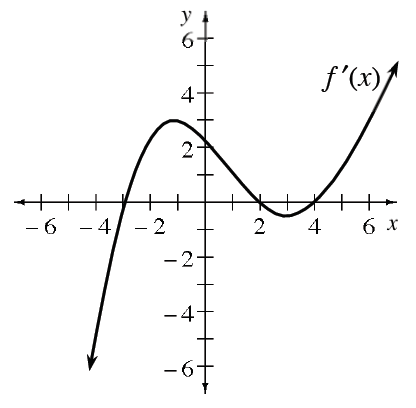### Home > APCALC > Chapter 4 > Lesson 4.4.2 > Problem4-153

4-153.

The graph of $y = f^\prime\left(x\right)$ is shown at right. Determine the intervals where $f$ is increasing, decreasing, concave up, and concave down.

We are looking at a graph of $f^\prime\left(x\right)$, but being asked about the graph of $f\left(x\right)$.
So think about what $f^\prime\left(x\right)$ tells us about $f\left(x\right).$

If $f^\prime\left(x\right)$ is positive, then $f\left(x\right)$ is increasing (and vice versa).
If $f^\prime\left(x\right)$ is increasing, then $f\left(x\right)$ is concave up (and vise versa).